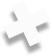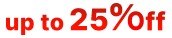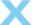We are committed to providing fast, efficient, and affordable software solutions that set new standards in the software development industry.# Pattern Example I

Below is an example of a commented pattern parsing an AVI file.

<?xml version="1.0" encoding="utf-8"?>

<!-- A pattern section. The pattern name is AVI File . -->

<template name="AVI File">

<!-- A template signature section. Alignment is 1. -->

<signature align="1">

<!-- A 4-byte signature at offset 0x00. -->

<field offset="0x00">52 49 46 46</field> <!-- ANSI: RIFF -->

<!-- A 4-byte signature at offset 0x08. -->

<field offset="0x08">41 56 49 20</field> <!-- ANSI: LIST -->

</signature>

<!-- A data section. Its name is AVI File . This is the main data section. It is not shown in the parsing tree as a section (its name is ignored). -->

<section name="AVI File">

<!-- The first 4 bytes are read and shown as an ANSI  string. -->

<field type="char" size="4" name="Signature: RIFF" var="signature"/>

<!--  The current position is moved to the beginning of the file. -->

<goto offset="-4"/>

<!-- The first 4 bytes in the file are read and shown as an unsigned integer. The internal variable signature gets the value of the field. -->

<field type="uint32" base="hex" name="Signature RIFF as unsigned integer in hex format" var="signature"/>

<!-- A test against the condition ( signature == RIFF ) -->

<if test="signature == 0x46464952"> <!-- ANSI: RIFF -->

<!-- The next 4 bytes are read and shown as an unsigned integer. The internal variable dataSize gets the value of  the field. -->

<field type="uint32" name="Size of the data in file" var="dataSize"/>

<!-- A new internal variable endOfFile is created and the expr field evaluates its value.-->

<setvar var="endOfFile" expr="offset + dataSize - 8"/>

<!-- The next 4 bytes is read and shown as an ANSI string. -->

<field type="char" size="4" name="File type"/>

<!-- A new section named DATA is created -->

<section name="DATA">

<!-- A new internal variable chunksOffset is created, the expr filed evaluating its value. This variable gets the absolute value of template offset. -->

<setvar var="chunksOffset" expr="start_position"/>

<!-- A loop is created. Its condition is set in the test field (while the endOfFile variable is greater then the current position.) -->

<repeat test="endOfFile > offset">

<!-- 4 bytes are read and shown as an ANSI string. -->

<field type="char" size="4" name="Signature"/>

<!-- The current position is moved backwards by 4 bytes. -->

<goto offset="-4"/>

<!-- The same 4 bytes are read and shown as an unsigned integer. The internal variable signature gets this value. -->

<field type="uint32" name="Signature as unsigned integer" var="signature"/>

<!-- A test against the condition ( signature == LIST ) -->

<if test="signature == 1414744396"> <!-- ANSI: LIST -->

<!-- The section is shown. Its name is LIST -->

<section name="LIST">

<!--  The current position is moved backward by 4 bytes. -->

<goto offset="-4"/>

<!-- The 4 bytes are read and shown as an unsigned hexadecimal integer. This field has the attributes offset and assigned-template . If the user double-clicks this field, the pattern will be invoked and the current pattern position will be moved to the address specified in as-offset .-->

<field type="uint32" base="hex" name="Signature LIST as unsigned integer in hex format" as-offset="start_position + offset - 4" assigned-template="AVI File LIST"/>

<!-- The next 4 bytes are read and shown as an unsigned integer. The listSize variable gets its value.  -->

<field type="uint32" name="Size of the data in the list" var="listSize"/>

<!-- The 4 bytes are read and shown as an ANSI string. -->

<field type="char" size="4" name="List type"/>

<!-- The current position is moved backward by 4 bytes. -->

<goto offset="-4"/>

<!-- The same 4 bytes are shown as an unsigned hexadecimal integer. The listType variable gets its value.-->

<field type="uint32" base="hex" name="List type as unsigned integer in hex format" var="listType"/>

<!-- A test against condition ( type == movi ) -->

<if test="listType == 0x69766f6d"> <!-- ANSI: movi -->

<!-- The chunksOffset variable gets the value evaluated in the expr attribute. -->

<setvar var="chunksOffset" expr="start_position + offset - 4"/>

</if>

<!-- The current position is moved to the address evaluated in the address attribute. -->

<goto address="offset + listSize - 4"/>

</section>

</if>

<!-- A test against condition ( signature == JUNK ) -->

<if test="signature == 1263424842"> <!-- ANSI: JUNK -->

<!--  The section is shown with the JUNK name. -->

<section name="JUNK">

<!-- The next 4 bytes are read and shown as an unsigned integer. The internal variable junkSize gets its value. -->

<field type="uint32" name="Size of the data of the junk" var="junkSize"/>

<!-- The current position is moved by junkSize bytes forward.  -->

<goto offset="junkSize"/>

</section>

</if>

<!-- A test against condition ( signature == idx1 ) -->

<if test="signature == 829973609"> <!-- ANSI: idx1 -->

<!--  The section is shown with the idx1 name. -->

<section name="idx1">

<!-- The next 4 bytes are read and shown as an unsigned integer. The internal variable idxSize gets its value. -->

<field type="uint32" name="Size of the data of the idx1" var="idxSize"/>

<!-- The section is shown with the First AVIINDEXENTRY name. -->

<section name="First AVIINDEXENTRY">

<!-- The 4 bytes are read and shown as an ANSI string. -->

<field type="char" size="4" name="Chunck id"/>

<!-- The next 4 bytes are read and shown as an unsigned hexadecimal integer. -->

<field type="uint32" base="hex" name="Flags"/>

<!-- The next 4 bytes are read ans shown as an unsigned hexadecimal integer. The offset attribute is evaluated for this field as a sum of  the chunksOffset variable and valued of this field.  -->

<field type="uint32" base="hex" name="Chunk offset" as-offset="chunksOffset + this"/>

<!-- The next 4 bytes are read and shown as an unsigned integer. -->

<field type="uint32" name="Chunk size"/>

</section>

<!-- The current position is moved by idxSiz bytes forward. -->

<goto offset="idxSize"/>

</section>

</if>

<!-- A test against the condition. &amp;&amp; is a logical AND ( && ) -->

<if test="signature != 1414744396 &amp;&amp; signature != 1263424842 &amp;&amp; signature != 829973609">

<!-- An empty section is shown. Its name is Unknown signature found -->

<section name="Unknown signature found">

</section>

<!-- The current position is moved to the address set in the endOfFile variable. -->

</if>

</repeat>

</section>

</if>

</section>

</template>